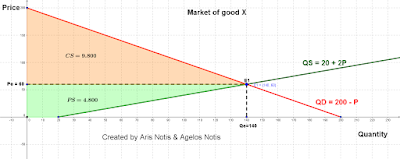## Sunday, 2 April 2017

The domestic market of good X is described by the equations: QD = 200 - P & Qs = 20 + 2P. The government decides to intervene in the market in order to increase its revenues by the imposition of 25% indirect tax per unit. What are the consequences to the stekeholders due to the imposition of the indirect tax?

The pre tax situation in the market is described by the accompanying graph.

After the imposition of the tax, producers received lower price per unit (Pp) than the price per unit which consumer pay (Pc). More specifically, Pp=0,75xPc. Thus, the cost of production is increased because Q's = 20 + 2x(0,75Pc) and the supply curve shifts upwards from Qs = 20 + 2P to Qs' = 20 + 1,5P.

The after tax situation in the market is represented diagrammatically below.

The conssequences to the different stakeholders are:
1. The consumer surplus decreases from \$9800 to \$8192.
2. The producer surplus decreases from \$4800 to \$3996.
3. Government revenues increases by \$2304.
4. Welfare loss equals to \$108.

## Wednesday, 29 March 2017

### Producer surplus

The domestic market of good X is described by the equations: Qd = 200 - P & Qs = 20 + 2P. Calculate the producer surplus.

Producer surplus (PS) is defined as the difference between what producers are willing to receive and what they actually receive from the sale of the good or service.

## Tuesday, 28 March 2017

### Consumer Surplus

The domestic market of good X is described by the equations: Qd = 200 - P & Qs = 20 + 2P. Calculate the consumer surplus.

Consumer surplus (CS) is defined as the difference between what consumers are willing and able to pay and what they actually pay in order to purchase the good or service.
In this case the CS = (140 x 140)/2 = \$9.800

## Sunday, 26 March 2017

### Equilibrium

The domestic market of good X is described by the equations: QD = 200 - P & Qs = 20 + 2P. Where QD & Qs is the quantity demanded and supplied in kg respectively and P is the price of the good in US dollars (\$). Calculate the price which consumers and producers are willing and able to pay and receive for the good X respectively.

The price equilibrium in the market of good X is where the demand of goods matches supply. It is calculated by the formula: QD = Qs or 200 - P = 20 + 2P or 3P = 180 or Pe = \$60. Thus, the quantity equilibrium is Qe = 200 - 60 = 140 units of product or Qe = 20 +  (2 x 60) = 140 units of product.
Total Surplus = Consumer Surplus + Producer Surplus = 9800 + 4800 = \$14600.

### Indirect tax (specific)

The domestic market of good X is described by the equations: QD = 200 - P & Qs = 20 + 2P. The government decides to intervene in the market in order to increase its revenues by the imposition of \$30 indirect tax per unit. What are the consequences to the stekeholders due to the imposition of the indirect tax?

After the imposition of the tax, producers receive lower price per unit (Pp) than the price per unit which consumer pay (Pc). More specifically, Pp = Pc - 30. Thus, the cost of production is increased because Q's = 20 + 2(Pc - 30) and the supply curve shifts upwards from Qs = 20 + 2P to Qs' = -40 + 2P.
The conssequences to the different stakeholders are:
1. The consumer surplus decreases from \$9800 to \$7200.
2. The producer surplus decreases from \$4800 to \$3500.
3. Government revenues increases by \$3600.
4. Welfare loss equals to \$300.

## Saturday, 25 March 2017

### Subsidy

The domestic market of a good X is described by the equations: Qd = 200 - P & Qs = 20 + 2P. The government decides to intervene in the market in order to protect this infant domestic industry by the imposition of \$30 subsidy. What are the consequences to the different stakeholders ?

The pre subsidy situation is illustrated above. After the subsidy, producers received greater price per unit (Pp) than the price per unit which consumer pay (Pc). More specifically, Pp = Pc + 30.
Thus, the cost of production is reduced because Q's = 20 + 2(Pc + 30) and the supply curve shifts to the right from Qs = 20 + 2P to Qs' = 80 + 2P.

The conssequences to the different stakeholders are:
1. The consumer surplus increases from \$9800 to \$12800. This means that the CS increases by \$3000.
2. The producer surplus increases from \$4800 to \$6300. This means that the PS increases by \$1500.
3. Government expenditure equals to \$4800.
4. Welfare loss equals to GVT expenditure - (CS+PS) = \$300.